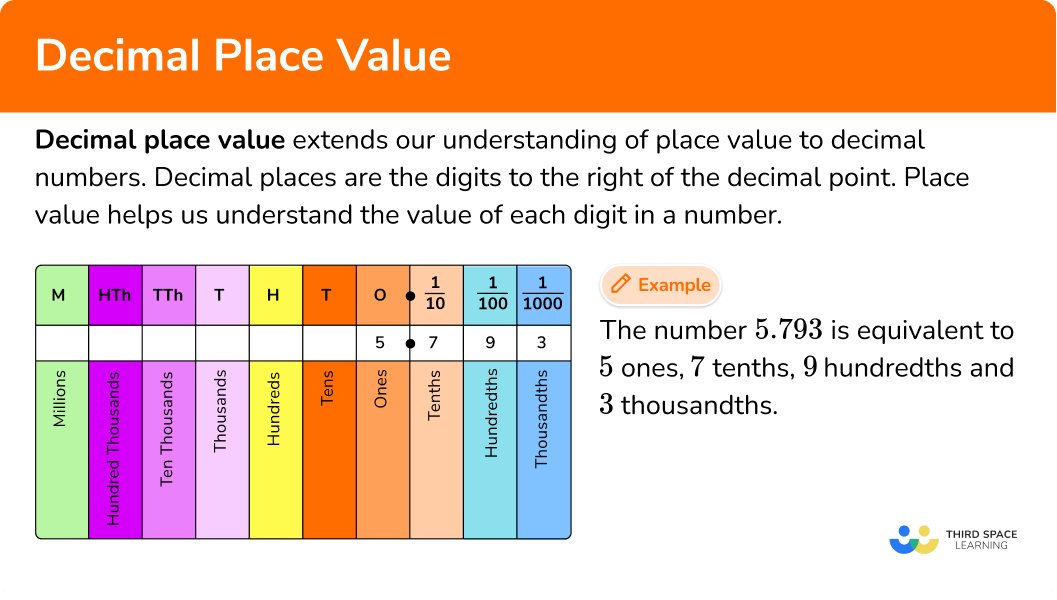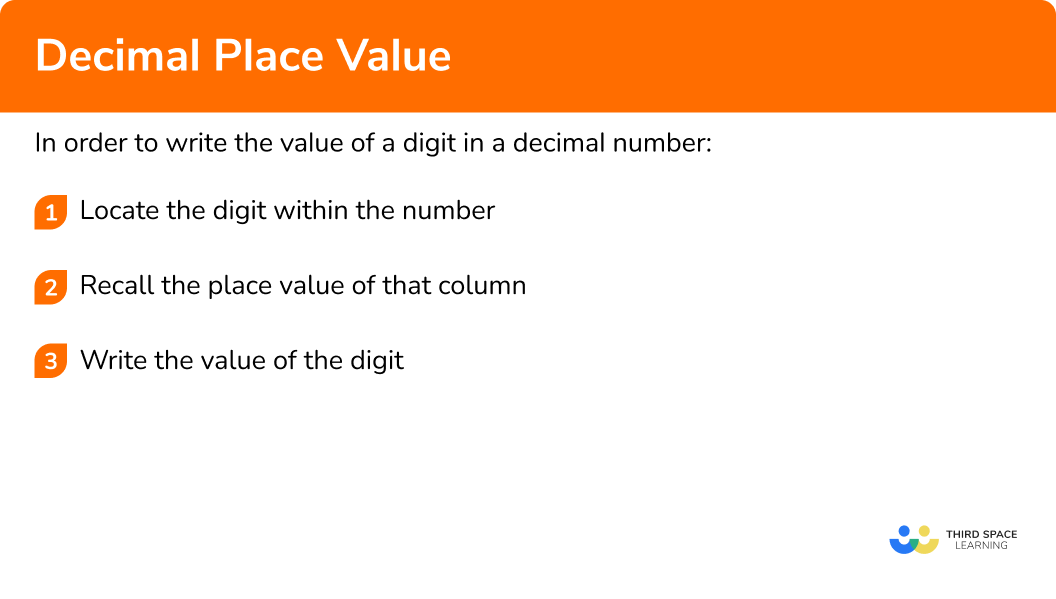GCSE Maths Number FDP Decimals

Decimal Place Value

# Decimal Place Value

Here we will learn about decimal place values, including what place value means for decimal numbers and how to use it.

There are also decimal place value worksheets based on Edexcel, AQA and OCR exam questions, along with further guidance on where to go next if you’re still stuck.

## What is decimal place value?

Decimal place value extends our understanding of place value to decimal numbers. Decimal places are the digits to the right of the decimal point.

The place value of a number helps us to understand the value of each digit in the number. To determine the value of each digit in a number we can use a place value chart or place value grid.

For example, let’s place the number 5.793 into the place value chart.

The digit 5 goes in the ones column.

The digit 7 goes in the tenths column.

The digit 9 goes in the hundredths column.

The digit 3 goes in the thousandths column.

This means that the number 5.793 is equivalent to 5 ones, 7 tenths, 9 hundredths and 3 thousandths.

### What is decimal place value?• Zero place holder

Sometimes we need to use zero as a placeholder in decimal numbers.

For example, the number 0.05 has zeros in the ones column and the tenths column. To indicate there are no ones and no tenths.

• Decimal fractions

Decimal fractions are fractions where the denominator is 10, \ 100, \ 1000 or another power of 10.

For example,

\frac{3}{10} \hspace{1cm} \frac{17}{100} \hspace{1cm} \frac{376}{1000} \hspace{1cm} \frac{19}{1000000}

Decimal fractions can also be used to write the value of the digits in a decimal number. This means that in the number 5.793 the digit 9 is worth 9 hundredths or 0.09 or \frac{9}{100}.

• Very small numbers

Decimal place values can be extended beyond tenths, hundredths and thousandths.

The decimal place value chart could be extended so there are more columns to the right. Powers of ten can be used to help write very small numbers.

• Ordering decimals

Decimal place value can help with ordering decimals.

For example,

Place these decimals in size order from smallest to largest.

0.06 \hspace{1cm} 0.1 \hspace{1cm} 0.007 \hspace{1cm} 0.0009

Let’s take each decimal and compare the place value of the digits.

The digit 9 in 0.0009 represents 9 ten-thousandths. This is the smallest value.

The digit 7 in 0.007 represents 7 thousandths. This is the next biggest value.

The digit 6 in 0.06 represents 6 hundreths. This is the next biggest value.

The digit 1 in 0.1 represents 1 tenth. This is the biggest value.

The decimals in order of size from smallest to largest will be:

0.0009 \hspace{1cm} 0.007 \hspace{1cm} 0.06 \hspace{1cm} 0.1

## How to write the value of a digit in a decimal number

In order to write the value of a digit in a decimal number:

1. Locate the digit within the number.
2. Recall the place value of that column.
3. Write the value of the digit.

### Explain how to write the value of a digit in a decimal number### Related lessons on decimals

Decimal place value is part of our series of lessons to support revision on decimals. You may find it helpful to start with the main decimals lesson for a summary of what to expect, or use the step by step guides below for further detail on individual topics. Other lessons in this series include:

## Decimal place value examples

### Example 1: tenths

Write the value of 3 in the number 4.392.

1. Locate the digit within the number.

2Recall the place value of that column.

The 3 is in the tenths place.

3Write the value of the digit.

The digit and the place value of the digit combine to give the value of the digit.

The value of the digit 3 in the number 4.392 is 3 tenths or 0.3 or as a decimal fraction \frac{3}{10}.

### Example 2: tenths

Write the value of 7 in the number 5.708.

Locate the digit within the number.

Recall the place value of that column.

Write the value of the digit.

### Example 3: hundredths

Write the value of 8 in the number 6.489.

Locate the digit within the number.

Recall the place value of that column.

Write the value of the digit.

### Example 4: hundredths

Write the value of 2 in the number 30.1289.

Locate the digit within the number.

Recall the place value of that column.

Write the value of the digit.

### Example 5: thousandths

Write the value of 1 in the number 35.941.

Locate the digit within the number.

Recall the place value of that column.

Write the value of the digit.

### Example 6: thousandths

Write the value of 9 in the number 835.219.

Locate the digit within the number.

Recall the place value of that column.

Write the value of the digit.

### Common misconceptions

• Thousands or thousandths

Numbers to the right of the decimal point are parts of a whole and so they each end with ‘ths’. Numbers to the left of the decimal point do not end in ‘ths’ as they are whole numbers.

### Practice decimal place value questions

1. Write the value of 1 in the number 7.129.

0.01one tenth\frac{1}{100}oneThe digit 1 is in the tenths column.

The value of the 1 is 1 tenth or 0.1 or \frac{1}{10}.

2. Write the value of 3 in the number 54.391.

3 hundreths30.03\frac{3}{10}The digit 3 is in the tenths column.

The value of the 3 is 3 tenths or 0.3 or \frac{3}{10}.

3. Write the value of 4 in the number 9.048.

0.0040.4\frac{4}{100}4 tenthsThe digit 4 is in the hundredths column.

The value of the 4 is 4 hundredths or 0.04 or \frac{4}{100}.

4. Write the value of 9 in the number 17.295.

\frac{9}{1000}9 thousandths0.90.09The digit 9 is in the hundredths column.

The value of the 9 is 9 hundredths or 0.09 or \frac{9}{100}.

5. Write the value of 2 in the number 197.8126.

\frac{2}{10000}2 ten thousandths0.022 thousandthsThe digit 2 is in the thousandths column.

The value of the 2 is 2 thousandths or 0.02 or \frac{2}{1000}.

6. Write the value of 6 in the number 438.1068.

\frac{6}{1000}6 hundreths0.0006\frac{6}{100}The digit 6 is in the thousandths column.

The value of the 6 is 6 thousandths or 0.06 or \frac{6}{1000}.

### Decimal place value GCSE questions

1. Use the number 56.973 to answer the following questions.

(a) What is the digit in the tens place?

(b) Write down the value of the digit 7.

(2 marks)

(a) 5

(1)

(b) 7 hundredths or 0.07 or \frac{7}{100}

(1)

2. (a) Write down the value of 3 in the number 2.319.

(b) Write down the value of 7 in the number 58.107.

(2 marks)

(a) 3 tenths or 0.3 or \frac{3}{10}

(1)

(b) 7 thousandths or 0.007 or \frac{7}{1000}

(1)

3. (a) Calculate 0.328 \div 10.

(b) Write down the value of 3 in the answer to part (a).

(2 marks)

(a) 0.0328

(1)

(b) 3 hundredths or 0.03 or \frac{3}{100}

(1)

4. (a) Ben says,

“the value of the digit 9 in 8.459 is 9000”.

(b) Callum says,

“0.009 is bigger than 0.08 because 9 is bigger than 8”.

(4 marks)

(a) No

(1)

Correct explanation involving thousandths

(1)

For example, Ben has said that the 9 is in the thousands column when he meant to say the thousandths column or 0.009.

(b) No

(1)

Correct explanation using the value of the digits 9 and 8.

(1)

For example, the 9 in 0.009 is worth \frac{9}{1000} or 9 thousandths.

The 8 in 0.08 is worth \frac{8}{100} or 8 hundreths.

So, 0.009 is actually smaller in value than 0.08.

5. In 2009 the world record for the 100 \ m sprint was 9.69 seconds.

Usain Bolt broke the world record for 100 \ m sprint.

Usain Bolt took 11 hundreths of a second off the previous world record.

Work out the time Usain Bolt ran, setting the new world record for the 100 \ m sprint.

(2 marks)

Subtracting 11 hundredths from 9.69.

(1)

9.58 seconds

(1)

## Learning checklist

You have now learned how to:

• Understand and use place value for decimals

## Still stuck?

Prepare your KS4 students for maths GCSEs success with Third Space Learning. Weekly online one to one GCSE maths revision lessons delivered by expert maths tutors.

Find out more about our GCSE maths tuition programme.